Home » How to create Beautiful, Interactive data visualizations using Plotly in R and Python?

# How to create Beautiful, Interactive data visualizations using Plotly in R and Python?

## Introduction

The greatest value of a picture is when it forces us to notice what we never expected to see.

―John Tukey

Data visualization is an art as well as a science. It takes constant practice and efforts to master the art of data visualization. I always keep exploring how to make my visualizations more interesting and informative. My main tool for creating these data visualizations had been ggplots. When I started using ggplots, I was amazed by its power. I felt like I was now an evolved story teller.

Then I realized that it is difficult to make interactive charts using ggplots. So, if you want to show something in 3 dimension, you can not look at it from various angles. So my exploration started again. One of the best alternatives, I found after spending hours was to learn D3.js. D3.js is a must know language if you really wish to excel in data visualization. Here, you can find a great resource to realize the power of D3.js and master it.

But I realized that D3.js is not as popular in data science community as it should have been, probably because it requires a different skill set (for ex. HTML, CSS and knowledge of JavaScript).

Today, I am going to tell you something which will change the way you perform data visualizations in the language / tool of your choice (R, Python, MATLAB, Perl, Julia, Arduino).

1. What is Plotly?
3. Steps for using Plotly
4. Setting up Data
5. Basic Visualizations
• Bar Charts
• Box Plots
• Scatter Plots
• Time Series Plots
• Heat Maps
• 3D Scatter Plots
• 3D Surfaces
7. Using plotly with ggplot2
8. Different versions of Plotly

## 1. What is Plotly?

Plotly is one of the finest data visualization tools available built on top of visualization library D3.js, HTML and CSS. It is created using Python and the Django framework.  One can choose to create interactive data visualizations online or use the libraries that plotly offers to create these visualizations in the language/ tool of choice. It is compatible with a number of languages/ tools: R, Python, MATLAB, Perl, Julia, Arduino.

Let’s have a look at some of the advantages and disadvantages of Plotly:

• It lets you create interactive visualizations built using D3.js without even having to know D3.js.
• It provides compatibility with number of different languages/ tools like R, Python, MATLAB, Perl, Julia, Arduino.
• Using plotly, interactive plots can easily be shared online with multiple people.
• Plotly can also be used by people with no technical background for creating interactive plots by uploading the data and using plotly GUI.
• Plotly is compatible with ggplots in R and Python.
• It allows to embed interactive plots in  projects or websites using iframes or html.
• The syntax for creating interactive plots using plotly is very simple as well.

• The plots made using plotly community version are always public and can be viewed by anyone.
• For plotly community version, there is an upper limit on the API calls per day.
• There are also limited  number of color Palettes available in community version which acts as an upper bound on the coloring options.

## 3. Steps for creating plots in Plotly.

Data visualization is an art with no hard and fast rules.

One simply should do what it takes to convey the message to the audience. Here is a series of typical steps for creating interactive plots using plotly

1. Getting the data to be used for creating visualization and preprocesisng it to convert it into the desired format.
2. Calling the plotly API in the language/ tool of your choice.
3. Creating the plot by specifying objectives like the data that is to be represented at each axis of the plot, most appropriate plot type (like histogram, boxplots, 3D surfaces), color of data points or line in the plot and other features. Here’s a generalized format for basic plotting in R and Python:

In R:

`plot_ly( x , y ,type,mode,color ,size )`

In Python:

`plotly.plotly( [plotly.graph_objs .type(x ,y ,mode , marker = dict(color ,size ))]`
• Where:
• size= values for same length as x, y and z that represents the size of datapoints or lines in plot.
• x =  values for x-axis
• y = values for y-axis
• type = to specify the plot that you want to create like “histogram”, “surface” , “box”, etc.
• mode = format in which you want data to be represented in the plot. Possible values are “markers”, “lines, “points”.
• color = values of same length as x, y and z that represents the color of datapoints or lines in plot.

4. Adding the layout fields like plot title axis title/ labels, axis title/ label fonts,  etc.

In R:

``layout(plot ,title , xaxis = list(title ,titlefont ), yaxis = list(title ,titlefont ))``

In Python:

`plotly.plotly.iplot( plot, plotly.graph_objs.Layout(title , xaxis = dict( title ,titlefont ), yaxis = dict( title ,titlefont)))`
• Where
• plot = the plotly object to be displayed
• title = string containing the title of the plot
• xaxis : title = title/ label for x-axis
• xaxis : titlefont = font for title/ label of x-axis
• yaxis : title = title/ label for y-axis
• yaxis : titlefont = font for title/ label of y-axis
1. Plotly also allows you to share the plots with someone else in various formats. For this, one needs to sign in to a plotly account. For sharing your plots you’ll need the following credentials: your username and your unique API key. Sharing the plots can be done as:

In R

``Sys.setenv("plotly_username"="XXXX")` `Sys.setenv("plotly_api_key"="YYYY")` `#To post the plots online` `plotly_POST(x = Plotting_Object)` `#To plot the last plot you created, simply use this.` `plotly_POST(x = last_plot(), filename = "XYZ")``

In Python

``#Setting plotly credentials` `plotly.tools.set_credentials_file(username=XXXX, api_key='YYYY’)` `#To post plots online` plotly`.plotly.plot(Plotting_Object)``

Since R and Python are two of the most popular languages among data scientists, I’ll be focusing on creating interactive visualizations using these two languages.

## 4. Setting up Data

For performing a wide range of interactive data visualizations, I’ll be using some of the publicly available datasets. You can follow the following code to get the datasets that I’ll be using during the course of this article :

### 4.1 Iris Data

#### In R

``#Loading iris dataset` `data(iris)` `#Structure of Iris dataset` `str(iris)` `## 'data.frame':    150 obs. of  5 variables:` `##  \$ Sepal.Length: num  5.1 4.9 4.7 4.6 5 5.4 4.6 5 4.4 4.9 ...` `##  \$ Sepal.Width : num  3.5 3 3.2 3.1 3.6 3.9 3.4 3.4 2.9 3.1 ...` `##  \$ Petal.Length: num  1.4 1.4 1.3 1.5 1.4 1.7 1.4 1.5 1.4 1.5 ...` `##  \$ Petal.Width : num  0.2 0.2 0.2 0.2 0.2 0.4 0.3 0.2 0.2 0.1 ...` `##  \$ Species     : Factor w/ 3 levels "setosa","versicolor",..: 1 1 1 1 1 1 1 1 1 1 ...``

#### In Python

``from sklearn import datasets` `import pandas as pd` `iris = datasets.load_iris()` `iris_df = pd.DataFrame(iris.data)` `iris_df.columns  = ['Sepal.Length','Sepal.Width','Petal.Length','Petal.Width']` `iris_df.columns` `iris_df['Species'] = iris.target` `iris_df['Species'] = iris_df['Species'].astype('category')` `iris_df.dtypes` `#Sepal.Length     float64` `#Sepal.Width      float64` `#Petal.Length     float64` `#Petal.Width      float64` `#Species         category` `#dtype: object` `iris_df['Species'].replace(0,'setosa',inplace=True)` `iris_df['Species'].replace(1,'versicolor',inplace=True)` `iris_df['Species'].replace(2,'virginica',inplace=True)``

### 4.2 International Airline Passengers Dataset

#### In R:

``#Loading the data` `data(AirPassengers)` `#Structure of International Airline Passengers Time series Dataset` `str(AirPassengers)` `#Time-Series [1:144] from 1949 to 1961: 112 118 132 129 121 135 148 148 136 119 ...``

#### In Python

You can get International airline passengers dataset here .

``#Loading the data` `airline_data = pd.read_csv('international-airline-passengers.csv')``

### 4.3 Volcano Dataset

#### In R

``#Loading the data` `data(volcano)` `#Checking dimensions` `dim(volcano)` `##  87 61``

#### In Python

You can get International airline passengers dataset here.

``#Loading the data` `volcano_data = pd.read_csv('volcano.csv')``

## 5. Basic Visualization

To get a good understanding of when you should use which plot, I’ll recommend you to check out this resource. Feel free to play around and explore these plots more. Here are a few things that you can try in the coming plots:

• hovering your mouse over the plot to view associated attributes
• selecting a particular region on the plot using your mouse to zoom
• resetting the axis
• rotating the 3D images

### 5.1 Histograms

You can view the interactive plot here.

#### In R

``library('plotly')` `#attaching the variables` `attach(iris)` `#plotting a histogram with Sepal.Length variable and storing it in hist` `hist<-plot_ly(x=Sepal.Length,type='histogram')` `#defining labels and title using layout()` `layout(hist,title = "Iris Dataset - Sepal.Length",` `xaxis = list(title = "Sepal.Length"),` `yaxis = list(title = "Count"))``

#### In Python

``import plotly.plotly as py` `import plotly.graph_objs as go` `data = [``go.Histogram(``x=iris.data[:,0]``)``]` `layout = go.Layout(` `title='Iris Dataset - Sepal.Length',` `xaxis=dict(``title='Sepal.Length'``),` `yaxis=dict(``title='Count'``)` `)` `fig = go.Figure(data=data, layout=layout)` `py.iplot(fig)``

### 5.2 Bar Charts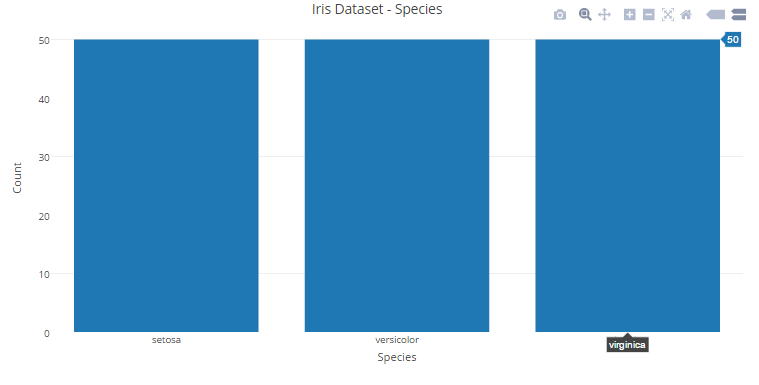You can view the interactive plot here.

#### In R

``#plotting a histogram with Species variable and storing it in bar_chart` `bar_chart<-plot_ly(x=Species,type='histogram')` `#defining labels and titile using layout()` `layout(bar_chart,title = "Iris Dataset - Species",` `xaxis = list(title = "Species"),` `yaxis = list(title = "Count"))``

#### In Python

``data = [``go.Bar(``x=['setosa','versicolor','virginica'],` `y=[iris_df.loc[iris_df['Species']=='setosa'].shape,iris_df.loc[iris_df['Species']=='versicolor'].shape,iris_df.loc[iris_df['Species']=='virginica'].shape]` `)``]` `layout = go.Layout(``title='Iris Dataset - Species',` `xaxis=dict(``title='Iris Dataset - Species'``),` `yaxis=dict(``title='Count'``)` `)` `fig = go.Figure(data=data, layout=layout)` `py.iplot(fig)``

### 5.3 Box Plots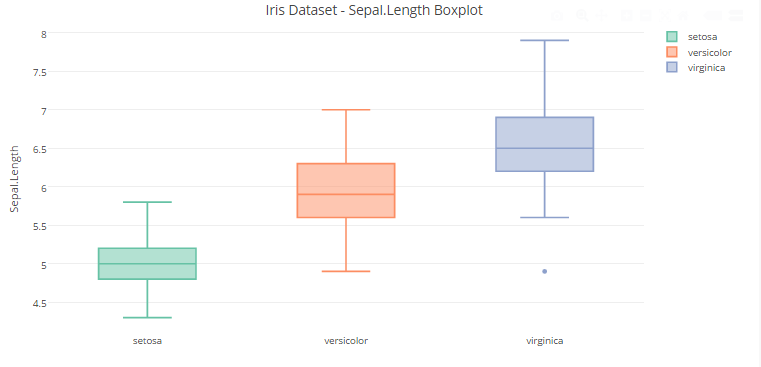You can view the interactive plot here.

#### In R

``#plotting a Boxplot with Sepal.Length variable and storing it in box_plot` `box_plot<-plot_ly(y=Sepal.Length,type='box',color=Species)` `#defining labels and title using layout()` `layout(box_plot,title = "Iris Dataset - Sepal.Length Boxplot",` `yaxis = list(title = "Sepal.Length"))``

#### In Python

``data = [go.Box(y=iris_df.loc[iris_df["Species"]=='setosa','Sepal.Length'],name='Setosa'),` `go.Box(y=iris_df.loc[iris_df["Species"]=='versicolor','Sepal.Length'],name='Versicolor'),` `go.Box(y=iris_df.loc[iris_df["Species"]=='virginica','Sepal.Length'],name='Virginica')]` `layout = go.Layout(``title='Iris Dataset - Sepal.Length Boxplot',` `yaxis=dict(``title='Sepal.Length'``)``)` `fig = go.Figure(data=data, layout=layout)` `py.iplot(fig)``

### 5.4 Scatter Plots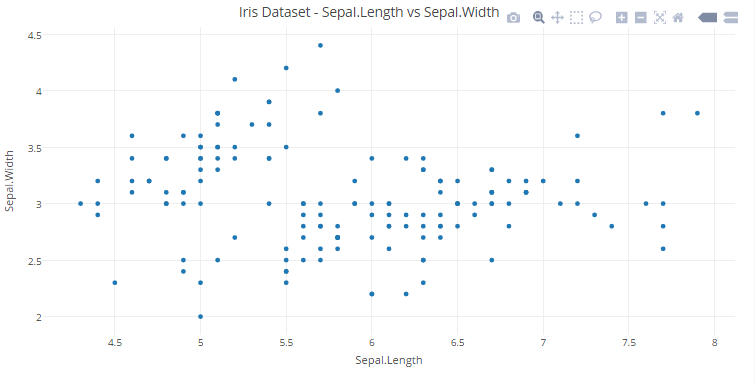You can view the interactive plot here.

#### In R

``#plotting a Scatter Plot with Sepal.Length and Sepal.Width variables and storing it in scatter_plot1` `scatter_plot1<-plot_ly(x=Sepal.Length,y=Sepal.Width,type='scatter',mode='markers')` `#defining labels and titile using layout()` `layout(scatter_plot1,title = "Iris Dataset - Sepal.Length vs Sepal.Width",` `xaxis = list(title = "Sepal.Length"),` `yaxis = list(title = "Sepal.Width"))``

#### In Python

``data = [go.Scatter(x = iris_df["Sepal.Length"],y = iris_df["Sepal.Width"],mode = 'markers')]` `layout = go.Layout(``title='Iris Dataset - Sepal.Length vs Sepal.Width',` `xaxis=dict(``title='Sepal.Length'``),` `yaxis=dict(``title='Sepal.Width'``)``)` `fig = go.Figure(data=data, layout=layout)` `py.iplot(fig)``
1. Let’s go a step further and add another dimension (Species) using color.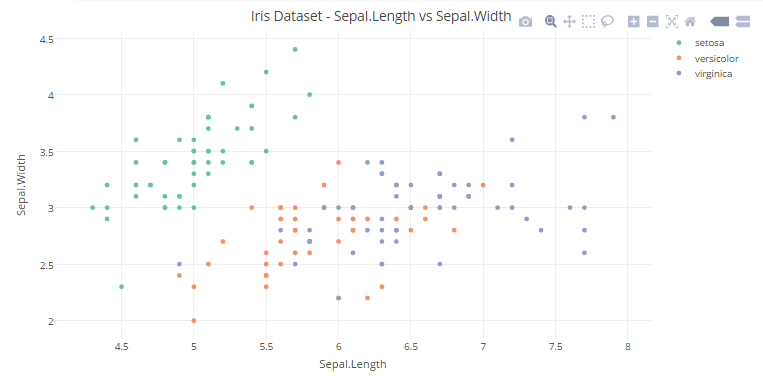You can view the interactive plot here.

#### In R

``#plotting a Scatter Plot with Sepal.Length and Sepal.Width variables with color representing the Species and storing it in scatter_plot12` `scatter_plot2<-plot_ly(x=Sepal.Length,y=Sepal.Width,type='scatter',mode='markers',color = Species)` `#defining labels and titile using layout()` `layout(scatter_plot2,title = "Iris Dataset - Sepal.Length vs Sepal.Width",` `xaxis = list(title = "Sepal.Length"),` `yaxis = list(title = "Sepal.Width"))``

#### In Python

``data = [go.Scatter(x = iris_df["Sepal.Length"],y = iris_df["Sepal.Width"],mode = 'markers', marker=dict(color = iris_df["Species"]))]` `layout = go.Layout(``title='Iris Dataset - Sepal.Length vs Sepal.Width',` `xaxis=dict(``title='Sepal.Length'``),` `yaxis=dict(``title='Sepal.Width'``)``)` `fig = go.Figure(data=data, layout=layout)` `py.iplot(fig)``

2. We can add another dimension (Petal Length) to the plot by using the size of each data point in the plot.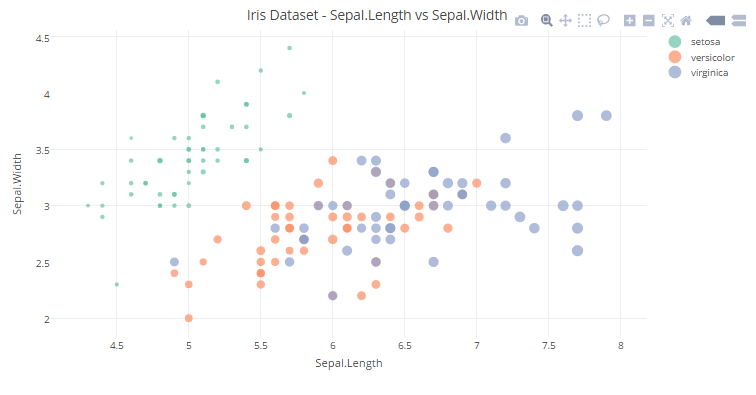You can view the interactive plot here.

``#plotting a Scatter Plot with Sepal.Length and Sepal.Width variables with color represneting the Species and size representing the Petal.Length. Then, storing it in scatter_plot3` `scatter_plot3<-plot_ly(x=Sepal.Length,y=Sepal.Width,type='scatter',mode='markers',color = Species,size=Petal.Length)` `#defining labels and titile using layout()` `layout(scatter_plot3,title = "Iris Dataset - Sepal.Length vs Sepal.Width",` `xaxis = list(title = "Sepal.Length"),` `yaxis = list(title = "Sepal.Width"))``

#### In Python

``data = [go.Scatter(x = iris_df["Sepal.Length"],y = iris_df["Sepal.Width"],mode = 'markers', marker=dict(color = iris_df["Species"],size=iris_df["Petal.Length"]))]` `layout = go.Layout(``title='Iris Dataset - Sepal.Length vs Sepal.Width',` `xaxis=dict(``title='Sepal.Length'``),` `yaxis=dict(``title='Sepal.Width'``)``)` `fig = go.Figure(data=data, layout=layout)` `py.iplot(fig)``

### 5.5 Time Series Plots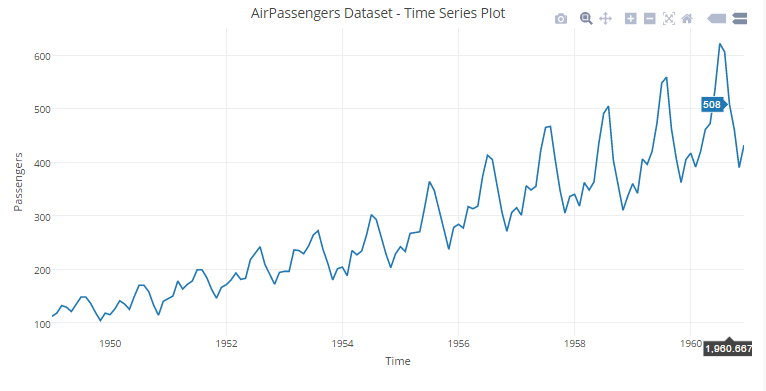You can view the interactive plot here.

#### In R

``#plotting a Boxplot with Sepal.Length variable and storing it in box_plot` `time_seies<-plot_ly(x=time(AirPassengers),y=AirPassengers,type="scatter",mode="lines")` `#defining labels and titile using layout()` `layout(time_seies,title = "AirPassengers Dataset - Time Series Plot",` `xaxis = list(title = "Time"),` `yaxis = list(title = "Passengers"))``

#### In Python

``data = [go.Scatter(x=airline_data.ix[:,0],y=airline_data.ix[:,1])]` `layout = go.Layout(` `title='AirPassengers Dataset - Time Series Plot',` `xaxis=dict(``title='Time'``),` `yaxis=dict(``title='Passengers'``)``)` `fig = go.Figure(data=data, layout=layout)` `py.iplot(fig)``

Till now, we have got a grasp of how plotly can be beneficial for basic visualizations. Now let’s shift gears and see plotly in action for advanced visualizations.

### 6.1 Heat Maps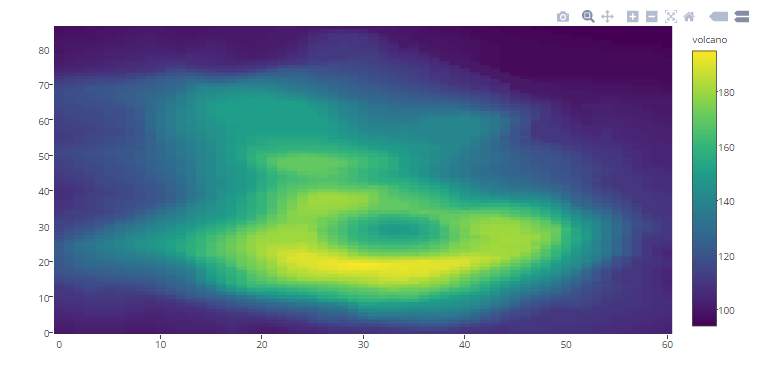You can view the interactive plot here.

#### In R

``plot_ly(z=~volcano,type="heatmap")``

#### In Python

``data = [go.Heatmap(z=volcano_data.as_matrix())]` `fig = go.Figure(data=data)` `py.iplot(fig)``

### 6.2 3D Scatter Plots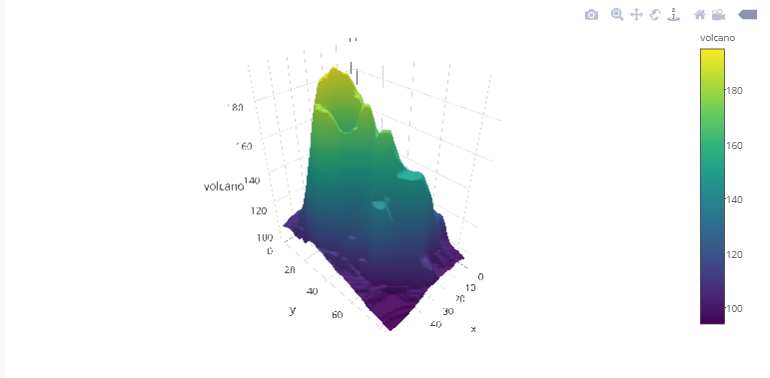You can view the interactive plot here.

#### In R

``#Plotting the Iris dataset in 3D` `plot_ly(x=Sepal.Length,y=Sepal.Width,z=Petal.Length,type="scatter3d",mode='markers',size=Petal.Width,color=Species)``

#### In Python

``data = [go.Scatter3d(x = iris_df["Sepal.Length"],y = iris_df["Sepal.Width"],z =  iris_df["Petal.Length"],mode = 'markers', marker=dict(color = iris_df["Species"],size=iris_df["Petal.Width"]))]` `fig = go.Figure(data=data)` `py.iplot(fig)``

### 6.3 3D Surfaces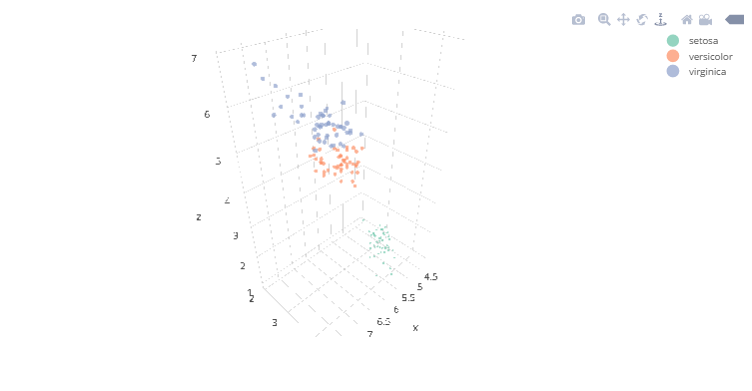You can view the interactive plot here.

#### In R

``#Plotting the volcano 3D surface` `plot_ly(z=~volcano,type="surface")``

#### In Python

``data = [go.Surface(z=volcano_data.as_matrix())]` `fig = go.Figure(data=data)` `py.iplot(fig)``

## 7. Using plotly with ggplot2

ggplot2 is one of the best visualization libraries out there. The best part about plotly is that it can add interactivity to ggplots and also ggplotly() which will further enhance the plots. For learning more about ggplot, you can check out this resource.

Let’s better understand it with an example in R.

``#Loading required libraries` `library('ggplot2')` `library('ggmap')` `#List of Countries for ICC T20 WC 2017` `ICC_WC_T20 <- c("Australia",` `"India",` `"South Africa",` `"New Zealand",` `"Sri Lanka",` `"England",` `"Bangladesh",` `"Pakistan",` `"West Indies",` `"Ireland",` `"Zimbabwe",` `"Afghanistan")` `#extract `geo location` of these countries` `countries <- geocode(ICC_WC_T20)` `#map longitude and latitude in separate variables` `nation.x <- countries\$lon` `nation.y <- countries\$lat` `#using ggplot to ``plot the world map` `mapWorld <- borders("world", colour="grey", fill="lightblue")` `#add data points to the world map` `q<-ggplot() + mapWorld + geom_point(aes(x=nation.x, y=nation.y) ,color="red", size=3)` `#Using `ggplotly`() of `ployly  to` add interactivity to `ggplot` objects.` ggplotly`(q) ``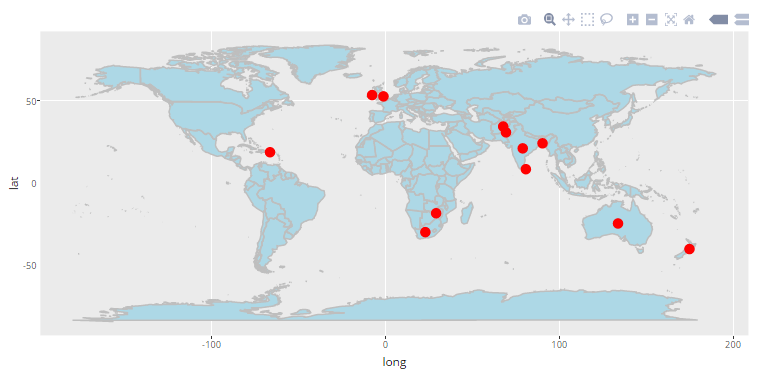You can view the interactive plot here.

## 8. Different versions of Plotly.

Plotly offers four different versions, namely:

1. Community
2. Personal
3. Professional
4. On-Premise

Each of these versions is differentiated based on pricing and features.  You can learn more about each of the versions here. The community version is free to get started and also provides decent capabilities. But one major drawback of community version is the inability to create private plots that to share online. If data security is a prominent challenge for an individual or organisation,  either of personal, professional or on-premise versions should be opted based upon the needs. For the above examples, I have used the community version.

## End Notes

After going through this article, you would have got a good grasp of how to create interactive plotly visualizations in R as well as Python. I personally use plotly a lot and find it really useful. Combining plotly with ggplots by using ggplotly() can give you the best visualizations in R or Python. But keep in mind that plotly is not limited to R and Python only, there a lot of other languages/ tools that it supports as well.

If you have any questions / doubts, do let me know in the comments below. If you enjoyed reading this article?  Do share your views in the comment section below.

### Learn, compete, hack and get hired!

You can also read this article on our Mobile APP•Akshat Tickoo says:

The plots are no longer visible. Your plotly subscription might need a revisit.

•Saurav Kaushik says:

Hi Akshat,

The problem has been fixed. Thanks for your concern.

•prakhar kushwah says:

very nicely explained sir.

got to know a new variety of visualization technique much simpler and easy to execute.

thanks a lot.

•Saurav Kaushik says:

Hi Prakhar,

I’m glad that you liked it.

•yash says:

Very good article Saurav. In fact last week I was asked to plot a timeseries data using plotly. But I refused and did it using ggplot2. But looking at this it seems plotly graphics are very good looking compared to the one’s done with ggplot2.
Can you please include a plot that shows placing text in between of the plots using a time series data.

•Saurav Kaushik says:

Hey Yash,

I also feel that plotly is comparable if not better than than ggplots. In fact, why now use the best of both by adding interactivity to your ggplots by using ggplotly() of plotly as I mentioned in this article.

Regarding your last query, You can simply add annotations at desired positions in the time series plotly graph. You can use these references:

https://plot.ly/r/text-and-annotations/

https://plot.ly/python/text-and-annotations/

•Lanre says:

Nice article and great job. please is there any site one can get a comprehensive tutorial on plotly. Thanks

•Saurav Kaushik says:

Hey Lanre,

Thank you. I believe, this article itself is sufficient to get started with plotly in whichever language you prefer: R or Python. In this article, one can learn from the generalized syntax for plotly in R and Python and follow the examples to get good grasp of possibilities for creating different plots using plotly. You can also refer to the official plotly site for more examples..

•Venkat says:

Hi Yash, great information! Thank you.

•Sambid Kumar Pradhan says:

Ahh!!! This is awesome , i always wanted to plot interactive worldmap ,but was limited was ggmap/ggplot

thanks Saurav

•Saurav Kaushik says:

Hi Sambid,

•Nageswara says:

Thank you for this awesome article. Is there any way we can get article in pdf format.

•Saurav Kaushik says:

Hi Nageswara,

Thank you but unfortunately the article might not be available in pdf format. My suggestion to you will be to bookmark this article instead for future reference.

•Nages says:

Hi Saurav,
Thanks for your quick response. Could you please let me know where do we can find bookmark option.
Regards,
Nages.

•Saurav Kaushik says:

Hi,

If you are using a laptop or computer, you can simply press Ctrl/Cmd + D on the webpage to bookmark it and if you are using your cellphone browser you might need to go through the options/menu to find the bookmark option once you are on this webpage.

•Nages says:

Thanks,Saurav,. I was thinking like some other way to do this.

•shivam says:

I want to ask that currently I m doing CSE and doing specialisation in BA but I don’t know how to start course in BA?
can you suggest me some options!!
Thank you..

•AVSu says:

Great article! It will be highly appreciated if you can share R code using plotly or ggplot2 to generate grouped vertical (or horizontal) bar charts similar to what was illustrated at http://www.jmp.com/support/help/Additional_Examples_of_the_Chart_Platform.shtml

Thanks!

•Bharat says:

Very Nice article Saurav. Do you have an example of using a background image in a plotly Plot in R ? I believe it is the ‘Images’ property of the layout. I just don’t know how best to use it.

•Dr.D.K.Samuel says:

Nice. Thanks

•Rahul K Gupta says:

Good Article. Well explained.
Basics to plotly visualization in Python.